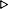ToC DocOverview CGDoc RelNotes Index PermutedIndex
Allegro CLANSI Common Lisp22 Printer22.4 Dictionary of Printer

Value Type:

Initial Value:
The initial value of *print-base* is 10. The initial value of *print-radix* is false.

Description:
*print-base* and *print-radix* control the printing of rationals. The value of *print-base* is called the current output base.

The value of *print-base* is the radix in which the printer will print rationals. For radices above 10, letters of the alphabet are used to represent digits above 9.

If the value of *print-radix* is true, the printer will print a radix specifier to indicate the radix in which it is printing a rational number. The radix specifier is always printed using lowercase letters. If *print-base* is 2, 8, or 16, then the radix specifier used is #b, #o, or #x, respectively. For integers, base ten is indicated by a trailing decimal point instead of a leading radix specifier; for ratios, #10r is used.

Examples:
``` (let ((*print-base* 24.) (*print-radix* t))
(print 23.))#24rN23
(setq *print-base* 10)10
(setq *print-radix* nil)NIL
(dotimes (i 35)
(let ((*print-base* (+ i 2)))           ;print the decimal number 40
(write 40)                            ;in each base from 2 to 36
(if (zerop (mod i 10)) (terpri) (format t " "))))1010001111 220 130 104 55 50 44 40 37 3431 2C 2A 28 26 24 22 20 1J 1I1H 1G 1F 1E 1D 1C 1B 1A 19 1817 16 15 14NIL
(dolist (pb '(2 3 8 10 16))
(let ((*print-radix* t)                 ;print the integer 10 and
(*print-base* pb))                ;the ratio 1/10 in bases 2,
(format t "~&~S  ~S~%" 10 1/10)))        ;3, 8, 10, 16#b1010  #b1/1010#3r101  #3r1/101#o12  #o1/1210.  #10r1/10#xA  #x1/ANIL
```

Affected By:
Might be bound by format, and write, write-to-string.